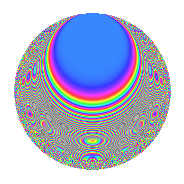# Properties

 Label 804.2.eLevel $804$ Weight $2$ Character orbit 804.e Rep. character $\chi_{804}(535,\cdot)$ Character field $\Q$ Dimension $68$ Newform subspaces $2$ Sturm bound $272$ Trace bound $3$

# Related objects

## Defining parameters

 Level: $$N$$ $$=$$ $$804 = 2^{2} \cdot 3 \cdot 67$$ Weight: $$k$$ $$=$$ $$2$$ Character orbit: $$[\chi]$$ $$=$$ 804.e (of order $$2$$ and degree $$1$$) Character conductor: $$\operatorname{cond}(\chi)$$ $$=$$ $$268$$ Character field: $$\Q$$ Newform subspaces: $$2$$ Sturm bound: $$272$$ Trace bound: $$3$$ Distinguishing $$T_p$$: $$7$$

## Dimensions

The following table gives the dimensions of various subspaces of $$M_{2}(804, [\chi])$$.

Total New Old
Modular forms 140 68 72
Cusp forms 132 68 64
Eisenstein series 8 0 8

## Trace form

 $$68q + 4q^{4} + 68q^{9} + O(q^{10})$$ $$68q + 4q^{4} + 68q^{9} - 12q^{10} - 8q^{14} + 4q^{16} + 8q^{21} - 16q^{22} - 12q^{24} - 68q^{25} + 20q^{26} - 32q^{29} + 4q^{36} + 24q^{37} - 36q^{40} + 92q^{49} + 28q^{56} - 24q^{60} - 4q^{62} + 52q^{64} - 68q^{68} + 24q^{73} - 64q^{76} - 16q^{77} + 68q^{81} + 52q^{82} - 16q^{84} + 12q^{86} - 56q^{88} - 12q^{90} - 92q^{92} - 8q^{93} + O(q^{100})$$

## Decomposition of $$S_{2}^{\mathrm{new}}(804, [\chi])$$ into newform subspaces

Label Dim. $$A$$ Field CM Traces $q$-expansion
$$a_2$$ $$a_3$$ $$a_5$$ $$a_7$$
804.2.e.a $$34$$ $$6.420$$ None $$0$$ $$-34$$ $$0$$ $$-4$$
804.2.e.b $$34$$ $$6.420$$ None $$0$$ $$34$$ $$0$$ $$4$$

## Decomposition of $$S_{2}^{\mathrm{old}}(804, [\chi])$$ into lower level spaces

$$S_{2}^{\mathrm{old}}(804, [\chi]) \cong$$ $$S_{2}^{\mathrm{new}}(268, [\chi])$$$$^{\oplus 2}$$

## Hecke characteristic polynomials

There are no characteristic polynomials of Hecke operators in the database